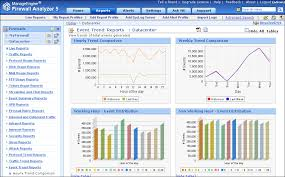Accounting

# Discuss and Analysis on Trend AnalysisGeneral objective of this article is to Discuss and Analysis on Trend Analysis. Here explain Trend Analysis in accounting perception. Trend analysis calculates the percentage change for example account over some time of two years or maybe more. Calculation system of Trend Analysis is calculate the number of the increase/(decrease) for the period by subtracting the earlier year from the later year. If the difference is negative, the change is a decrease and if the difference is positive, it’s an increase. Divide the change by the earlier year’s balance. The end result is the percentage change.